# How To Build a Deep Learning Model to Predict Employee Retention Using Keras and TensorFlow

Published on April 25, 2019 · Updated on April 4, 2022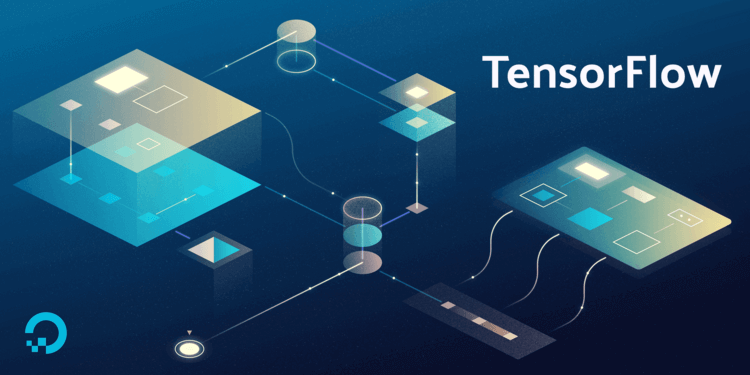The author selected Girls Who Code to receive a donation as part of the Write for DOnations program.

### Introduction

Keras is a neural network API that is written in Python. It runs on top of TensorFlow, CNTK, or Theano. It is a high-level abstraction of these deep learning frameworks and therefore makes experimentation faster and easier. Keras is modular, which means implementation is seamless as developers can quickly extend models by adding modules.

TensorFlow is an open-source software library for machine learning. It works efficiently with computation involving arrays; so it’s a great choice for the model you’ll build in this tutorial. Furthermore, TensorFlow allows for the execution of code on either CPU or GPU, which is a useful feature especially when you’re working with a massive dataset.

In this tutorial, you’ll build a deep learning model that will predict the probability of an employee leaving a company. Retaining the best employees is an important factor for most organizations. To build your model, you’ll use this dataset available at Kaggle, which has features that measure employee satisfaction in a company. To create this model, you’ll use the Keras sequential layer to build the different layers for the model.

## Prerequisites

Before you begin this tutorial you’ll need the following:

## Step 1 — Data Pre-processing

Data Pre-processing is necessary to prepare your data in a manner that a deep learning model can accept. If there are categorical variables in your data, you have to convert them to numbers because the algorithm only accepts numerical figures. A categorical variable represents quantitive data represented by names. In this step, you’ll load in your dataset using `pandas`, which is a data manipulation Python library.

Before you begin data pre-processing, you’ll activate your environment and ensure you have all the necessary packages installed to your machine. It’s advantageous to use `conda` to install `keras` and `tensorflow` since it will handle the installation of any necessary dependencies for these packages, and ensure they are compatible with `keras` and `tensorflow`. In this way, using the Anaconda Python distribution is a good choice for data science related projects.

Move into the environment you created in the prerequisite tutorial:

``````conda activate my_env

``````

Run the following command to install `keras` and `tensorflow`:

``````conda install tensorflow keras

``````

Now, open Jupyter Notebook to get started. Jupyter Notebook is opened by typing the following command on your terminal:

``````jupyter notebook

``````

Note: If you’re working from a remote server, you’ll need to use SSH tunneling to access your notebook. Please revisit step 2 of the prerequisite tutorial for detailed on instructions on setting up SSH tunneling. You can use the following command from your local machine to initiate your SSH tunnel:

``````ssh -L 8888:localhost:8888 your_username@your_server_ip

``````

After accessing Jupyter Notebook, click on the anaconda3 file, and then click New at the top of the screen, and select Python 3 to load a new notebook.

Now, you’ll import the required modules for the project and then load the dataset in a notebook cell. You’ll load in the `pandas` module for manipulating your data and `numpy` for converting the data into `numpy` arrays. You’ll also convert all the columns that are in string format to numerical values for your computer to process.

Insert the following code into a notebook cell and then click Run:

``````import pandas as pd
import numpy as np
``````

You’ve imported `numpy` and `pandas`. You then used `pandas` to load in the dataset for the model.

You can get a glimpse at the dataset you’re working with by using `head()`. This is a useful function from `pandas` that allows you to view the first five records of your dataframe. Add the following code to a notebook cell and then run it:

``````df.head()
``````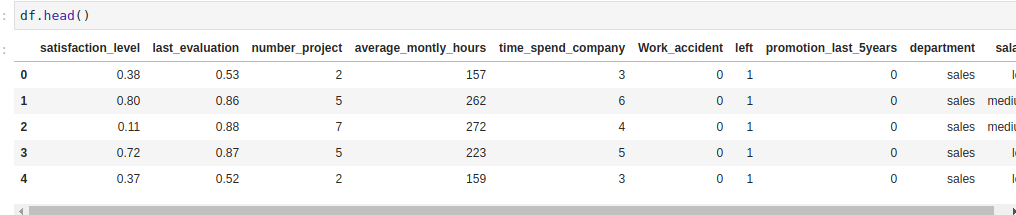You’ll now proceed to convert the categorical columns to numbers. You do this by converting them to dummy variables. Dummy variables are usually ones and zeros that indicate the presence or absence of a categorical feature. In this kind of situation, you also avoid the dummy variable trap by dropping the first dummy.

Note: The dummy variable trap is a situation whereby two or more variables are highly correlated. This leads to your model performing poorly. You, therefore, drop one dummy variable to always remain with N-1 dummy variables. Any of the dummy variables can be dropped because there is no preference as long as you remain with N-1 dummy variables. An example of this is if you were to have an on/off switch. When you create the dummy variable you shall get two columns: an `on` column and an `off` column. You can drop one of the columns because if the switch isn’t on, then it is off.

Insert this code in the next notebook cell and execute it:

``````feats = ['department','salary']
df_final = pd.get_dummies(df,columns=feats,drop_first=True)
``````

`feats = ['department','salary']` defines the two columns for which you want to create dummy variables. `pd.get_dummies(df,columns=feats,drop_first=True)` will generate the numerical variables that your employee retention model requires. It does this by converting the `feats` that you define from categorical to numerical variables.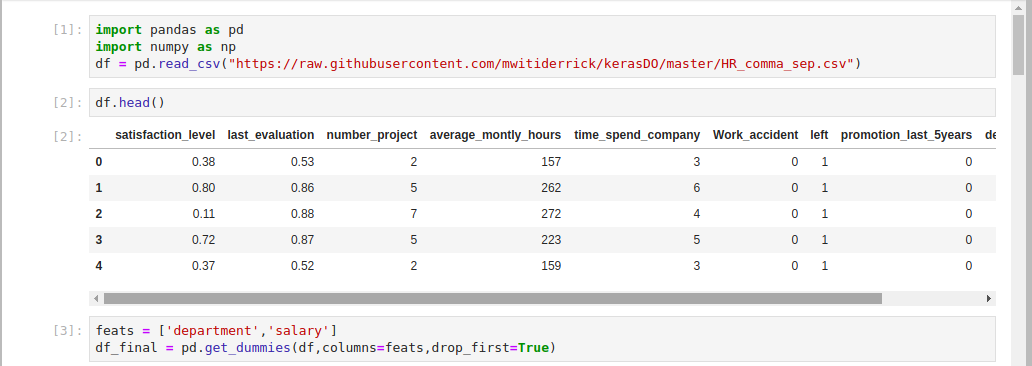You’ve loaded in the dataset and converted the salary and department columns into a format the `keras` deep learning model can accept. In the next step, you will split the dataset into a training and testing set.

## Step 2 — Separating Your Training and Testing Datasets

You’ll use `scikit-learn` to split your dataset into a training and a testing set. This is necessary so you can use part of the employee data to train the model and a part of it to test its performance. Splitting a dataset in this way is a common practice when building deep learning models.

It is important to implement this split in the dataset so the model you build doesn’t have access to the testing data during the training process. This ensures that the model learns only from the training data, and you can then test its performance with the testing data. If you exposed your model to testing data during the training process then it would memorize the expected outcomes. Consequently, it would fail to give accurate predictions on data that it hasn’t seen.

You’ll start by importing the `train_test_split` module from the `scikit-learn` package. This is the module that will provide the splitting functionality. Insert this code in the next notebook cell and run:

``````from sklearn.model_selection import train_test_split
``````

With the `train_test_split` module imported, you’ll use the `left` column in your dataset to predict if an employee will leave the company. Therefore, it is essential that your deep learning model doesn’t come into contact with this column. Insert the following into a cell to drop the `left` column:

``````X = df_final.drop(['left'],axis=1).values
y = df_final['left'].values
``````

Your deep learning model expects to get the data as arrays. Therefore you use `numpy` to convert the data to `numpy` arrays with the `.values` attribute.

You’re now ready to convert the dataset into a testing and training set. You’ll use 70% of the data for training and 30% for testing. The training ratio is more than the testing ratio because you’ll need to use most of the data for the training process. If desired, you can also experiment with a ratio of 80% for the training set and 20% for the testing set.

Now add this code to the next cell and run to split your training and testing data to the specified ratio:

``````X_train, X_test, y_train, y_test = train_test_split(X, y, test_size=0.3)
``````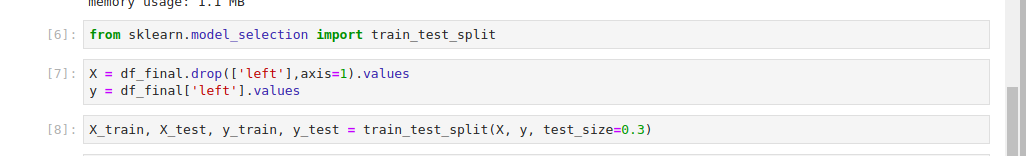You have now converted the data into the type that Keras expects it to be in (`numpy` arrays), and your data is split into a training and testing set. You’ll pass this data to the `keras` model later in the tutorial. Beforehand you need to transform the data, which you’ll complete in the next step.

## Step 3 — Transforming the Data

When building deep learning models it is usually good practice to scale your dataset in order to make the computations more efficient. In this step, you’ll scale the data using the `StandardScaler`; this will ensure that your dataset values have a mean of zero and a unit variable. This transforms the dataset to be normally distributed. You’ll use the `scikit-learn` `StandardScaler` to scale the features to be within the same range. This will transform the values to have a mean of 0 and a standard deviation of 1. This step is important because you’re comparing features that have different measurements; so it is typically required in machine learning.

To scale the training set and the test set, add this code to the notebook cell and run it:

``````from sklearn.preprocessing import StandardScaler
sc = StandardScaler()
X_train = sc.fit_transform(X_train)
X_test = sc.transform(X_test)
``````

Here, you start by importing the `StandardScaler` and calling an instance of it. You then use its `fit_transform` method to scale the training and testing set.

You have scaled all your dataset features to be within the same range. You can start building the artificial neural network in the next step.

## Step 4 — Building the Artificial Neural Network

Now you will use `keras` to build the deep learning model. To do this, you’ll import `keras`, which will use `tensorflow` as the backend by default. From `keras`, you’ll then import the `Sequential` module to initialize the artificial neural network. An artificial neural network is a computational model that is built using inspiration from the workings of the human brain. You’ll import the `Dense` module as well, which will add layers to your deep learning model.

When building a deep learning model you usually specify three layer types:

• The input layer is the layer to which you’ll pass the features of your dataset. There is no computation that occurs in this layer. It serves to pass features to the hidden layers.
• The hidden layers are usually the layers between the input layer and the output layer—and there can be more than one. These layers perform the computations and pass the information to the output layer.
• The output layer represents the layer of your neural network that will give you the results after training your model. It is responsible for producing the output variables.

To import the `Keras`, `Sequential`, and `Dense` modules, run the following code in your notebook cell:

``````import keras
from keras.models import Sequential
from keras.layers import Dense
``````

You’ll use `Sequential` to initialize a linear stack of layers. Since this is a classification problem, you’ll create a classifier variable. A classification problem is a task where you have labeled data and would like to make some predictions based on the labeled data. Add this code to your notebook to create a classifier variable:

``````classifier = Sequential()
``````

You’ve used `Sequential` to initialize the classifier.

``````classifier.add(Dense(9, kernel_initializer = "uniform",activation = "relu", input_dim=18))
``````

You add layers using the `.add()` function on your classifier and specify some parameters:

• The first parameter is the number of nodes that your network should have. The connection between different nodes is what forms the neural network. One of the strategies to determine the number of nodes is to take the average of the nodes in the input layer and the output layer.

• The second parameter is the `kernel_initializer.` When you fit your deep learning model the weights will be initialized to numbers close to zero, but not zero. To achieve this you use the uniform distribution initializer. `kernel_initializer` is the function that initializes the weights.

• The third parameter is the `activation` function. Your deep learning model will learn through this function. There are usually linear and non-linear activation functions. You use the `relu` activation function because it generalizes well on your data. Linear functions are not good for problems like these because they form a straight line.

• The last parameter is `input_dim`, which represents the number of features in your dataset.

Now you’ll add the output layer that will give you the predictions:

``````classifier.add(Dense(1, kernel_initializer = "uniform",activation = "sigmoid"))
``````

The output layer takes the following parameters:

• The number of output nodes. You expect to get one output: if an employee leaves the company. Therefore you specify one output node.

• For `kernel_initializer` you use the `sigmoid` activation function so that you can get the probability that an employee will leave. In the event that you were dealing with more than two categories, you would use the `softmax` activation function, which is a variant of the `sigmoid` activation function.

Next, you’ll apply a gradient descent to the neural network. This is an optimization strategy that works to reduce errors during the training process. Gradient descent is how randomly assigned weights in a neural network are adjusted by reducing the cost function, which is a measure of how well a neural network performs based on the output expected from it.

The aim of a gradient descent is to get the point where the error is at its least. This is done by finding where the cost function is at its minimum, which is referred to as a local minimum. In gradient descent, you differentiate to find the slope at a specific point and find out if the slope is negative or positive—you’re descending into the minimum of the cost function. There are several types of optimization strategies, but you’ll use a popular one known as `adam` in this tutorial.

``````classifier.compile(optimizer= "adam",loss = "binary_crossentropy",metrics = ["accuracy"])
``````

Applying gradient descent is done via the `compile` function that takes the following parameters:

• `optimizer` is the gradient descent.
• `loss` is a function that you’ll use in the gradient descent. Since this is a binary classification problem you use the `binary_crossentropy` `loss` function.
• The last parameter is the `metric` that you’ll use to evaluate your model. In this case, you’d like to evaluate it based on its accuracy when making predictions.

You’re ready to fit your classifier to your dataset. Keras makes this possible via the `.fit()` method. To do this, insert the following code into your notebook and run it in order to fit the model to your dataset:

``````classifier.fit(X_train, y_train, batch_size = 10, epochs = 1)
``````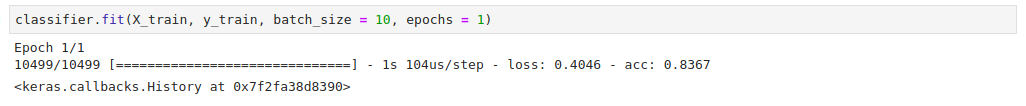The `.fit()` method takes a couple of parameters:

• The first parameter is the training set with the features.

• The second parameter is the column that you’re making the predictions on.

• The `batch_size` represents the number of samples that will go through the neural network at each training round.

• `epochs` represents the number of times that the dataset will be passed via the neural network. The more epochs the longer it will take to run your model, which also gives you better results.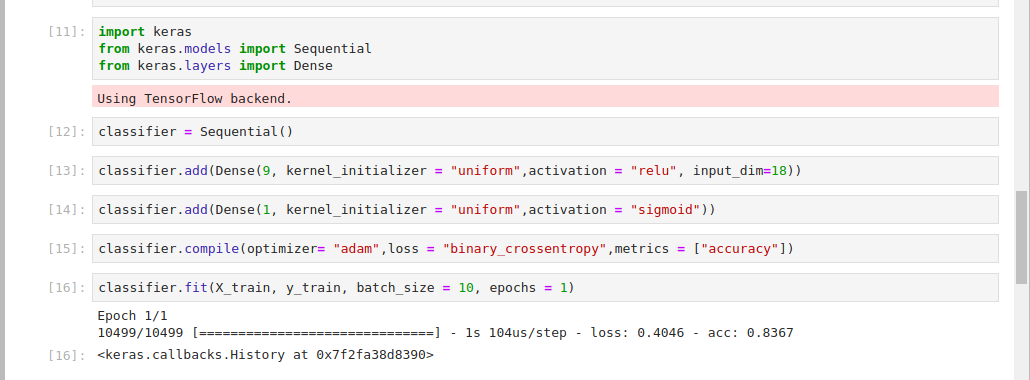You’ve created your deep learning model, compiled it, and fitted it to your dataset. You’re ready to make some predictions using the deep learning model. In the next step, you’ll start making predictions with the dataset that the model hasn’t yet seen.

## Step 5 — Running Predictions on the Test Set

To start making predictions, you’ll use the testing dataset in the model that you’ve created. Keras enables you to make predictions by using the `.predict()` function.

Insert the following code in the next notebook cell to begin making predictions:

``````y_pred = classifier.predict(X_test)
``````

Since you’ve already trained the classifier with the training set, this code will use the learning from the training process to make predictions on the test set. This will give you the probabilities of an employee leaving. You’ll work with a probability of 50% and above to indicate a high chance of the employee leaving the company.

Enter the following line of code in your notebook cell in order to set this threshold:

``````y_pred = (y_pred > 0.5)
``````

You’ve created predictions using the predict method and set the threshold for determining if an employee is likely to leave. To evaluate how well the model performed on the predictions, you will next use a confusion matrix.

## Step 6 — Checking the Confusion Matrix

In this step, you will use a confusion matrix to check the number of correct and incorrect predictions. A confusion matrix, also known as an error matrix, is a square matrix that reports the number of true positives(tp), false positives(fp), true negatives(tn), and false negatives(fn) of a classifier.

• A true positive is an outcome where the model correctly predicts the positive class (also known as sensitivity or recall).
• A true negative is an outcome where the model correctly predicts the negative class.
• A false positive is an outcome where the model incorrectly predicts the positive class.
• A false negative is an outcome where the model incorrectly predicts the negative class.

To achieve this you’ll use a confusion matrix that `scikit-learn` provides.

Insert this code in the next notebook cell to import the `scikit-learn` confusion matrix:

``````from sklearn.metrics import confusion_matrix
cm = confusion_matrix(y_test, y_pred)
cm
``````

The confusion matrix output means that your deep learning model made `3305 + 375` correct predictions and `106 + 714` wrong predictions. You can calculate the accuracy with: `(3305 + 375) / 4500`. The total number of observations in your dataset is 4500. This gives you an accuracy of 81.7%. This is a very good accuracy rate since you can achieve at least 81% correct predictions from your model.

``````Outputarray([[3305,  106],
[ 714,  375]])
``````

You’ve evaluated your model using the confusion matrix. Next, you’ll work on making a single prediction using the model that you have developed.

## Step 7 — Making a Single Prediction

In this step you’ll make a single prediction given the details of one employee with your model. You will achieve this by predicting the probability of a single employee leaving the company. You’ll pass this employee’s features to the `predict` method. As you did earlier, you’ll scale the features as well and convert them to a `numpy` array.

To pass the employee’s features, run the following code in a cell:

``````new_pred = classifier.predict(sc.transform(np.array([[0.26,0.7 ,3., 238., 6., 0.,0.,0.,0., 0.,0.,0.,0.,0.,1.,0., 0.,1.]])))
``````

These features represent the features of a single employee. As shown in the dataset in step 1, these features represent: satisfaction level, last evaluation, number of projects, and so on. As you did in step 3, you have to transform the features in a manner that the deep learning model can accept.

Add a threshold of 50% with the following code:

``````new_pred = (new_pred > 0.5)
new_pred
``````

This threshold indicates that where the probability is above 50% an employee will leave the company.

You can see in your output that the employee won’t leave the company:

``````Outputarray([[False]])
``````

You might decide to set a lower or higher threshold for your model. For example, you can set the threshold to be 60%:

``````new_pred = (new_pred > 0.6)
new_pred
``````

This new threshold still shows that the employee won’t leave the company:

``````Outputarray([[False]])
``````

In this step, you have seen how to make a single prediction given the features of a single employee. In the next step, you will work on improving the accuracy of your model.

## Step 8 — Improving the Model Accuracy

If you train your model many times you’ll keep getting different results. The accuracies for each training have a high variance. In order to solve this problem, you’ll use K-fold cross-validation. Usually, K is set to 10. In this technique, the model is trained on the first 9 folds and tested on the last fold. This iteration continues until all folds have been used. Each of the iterations gives its own accuracy. The accuracy of the model becomes the average of all these accuracies.

`keras` enables you to implement K-fold cross-validation via the `KerasClassifier` wrapper. This wrapper is from `scikit-learn` cross-validation. You’ll start by importing the `cross_val_score` cross-validation function and the `KerasClassifier`. To do this, insert and run the following code in your notebook cell:

``````from keras.wrappers.scikit_learn import KerasClassifier
from sklearn.model_selection import cross_val_score
``````

To create the function that you will pass to the `KerasClassifier`, add this code to the next cell:

``````def make_classifier():
classifier = Sequential()
classifier.add(Dense(9, kernel_initializer = "uniform", activation = "relu", input_dim=18))
classifier.add(Dense(1, kernel_initializer = "uniform", activation = "sigmoid"))
classifier.compile(optimizer= "adam",loss = "binary_crossentropy",metrics = ["accuracy"])
return classifier
``````

Here, you create a function that you’ll pass to the `KerasClassifier`—the function is one of the arguments that the classifier expects. The function is a wrapper of the neural network design that you used earlier. The passed parameters are also similar to the ones used earlier in the tutorial. In the function, you first initialize the classifier using `Sequential()`, you then use `Dense` to add the input and output layer. Finally, you compile the classifier and return it.

To pass the function you’ve built to the `KerasClassifier`, add this line of code to your notebook:

``````classifier = KerasClassifier(build_fn = make_classifier, batch_size=10, nb_epoch=1)
``````

The `KerasClassifier` takes three arguments:

• `build_fn`: the function with the neural network design
• `batch_size`: the number of samples to be passed via the network in each iteration
• `nb_epoch`: the number of epochs the network will run

Next, you apply the cross-validation using Scikit-learn’s `cross_val_score`. Add the following code to your notebook cell and run it:

``````accuracies = cross_val_score(estimator = classifier,X = X_train,y = y_train,cv = 10,n_jobs = -1)
``````

This function will give you ten accuracies since you have specified the number of folds as 10. Therefore, you assign it to the accuracies variable and later use it to compute the mean accuracy. It takes the following arguments:

• `estimator`: the classifier that you’ve just defined
• `X`: the training set features
• `y`: the value to be predicted in the training set
• `cv`: the number of folds
• `n_jobs`: the number of CPUs to use (specifying it as -1 will make use of all the available CPUs)

Now you have applied the cross-validation, you can compute the mean and variance of the accuracies. To achieve this, insert the following code into your notebook:

``````mean = accuracies.mean()
mean
``````

In your output you’ll see that the mean is 83%:

``````Output0.8343617910685696
``````

To compute the variance of the accuracies, add this code to the next notebook cell:

``````variance = accuracies.var()
variance
``````

You see that the variance is 0.00109. Since the variance is very low, it means that your model is performing very well.

``````Output0.0010935021002275425
``````

You’ve improved your model’s accuracy by using K-Fold cross-validation. In the next step, you’ll work on the overfitting problem.

## Step 9 — Adding Dropout Regularization to Fight Over-Fitting

Predictive models are prone to a problem known as overfitting. This is a scenario whereby the model memorizes the results in the training set and isn’t able to generalize on data that it hasn’t seen. Typically you observe overfitting when you have a very high variance on accuracies. To help fight over-fitting in your model, you will add a layer to your model.

In neural networks, dropout regularization is the technique that fights overfitting by adding a `Dropout` layer in your neural network. It has a `rate` parameter that indicates the number of neurons that will deactivate at each iteration. The process of deactivating nerurons is usually random. In this case, you specify 0.1 as the rate meaning that 1% of the neurons will deactivate during the training process. The network design remains the same.

To add your `Dropout` layer, add the following code to the next cell:

``````from keras.layers import Dropout

classifier = Sequential()
classifier.add(Dense(9, kernel_initializer = "uniform", activation = "relu", input_dim=18))
classifier.add(Dense(1, kernel_initializer = "uniform", activation = "sigmoid"))
classifier.compile(optimizer= "adam",loss = "binary_crossentropy",metrics = ["accuracy"])
``````

You have added a `Dropout` layer between the input and output layer. Having set a dropout rate of 0.1 means that during the training process 15 of the neurons will deactivate so that the classifier doesn’t overfit on the training set. After adding the `Dropout` and output layers you then compiled the classifier as you have done previously.

You worked to fight over-fitting in this step with a `Dropout` layer. Next, you’ll work on further improving the model by tuning the parameters you used while creating the model.

## Step 10 — Hyperparameter Tuning

Grid search is a technique that you can use to experiment with different model parameters in order to obtain the ones that give you the best accuracy. The technique does this by trying different parameters and returning those that give the best results. You’ll use grid search to search for the best parameters for your deep learning model. This will help in improving model accuracy. `scikit-learn` provides the `GridSearchCV` function to enable this functionality. You will now proceed to modify the `make_classifier` function to try out different parameters.

Add this code to your notebook to modify the `make_classifier` function so you can test out different optimizer functions:

``````from sklearn.model_selection import GridSearchCV
def make_classifier(optimizer):
classifier = Sequential()
classifier.add(Dense(9, kernel_initializer = "uniform", activation = "relu", input_dim=18))
classifier.add(Dense(1, kernel_initializer = "uniform", activation = "sigmoid"))
classifier.compile(optimizer= optimizer,loss = "binary_crossentropy",metrics = ["accuracy"])
return classifier
``````

You have started by importing `GridSearchCV`. You have then made changes to the `make_classifier` function so that you can try different optimizers. You’ve initialized the classifier, added the input and output layer, and then compiled the classifier. Finally, you have returned the classifier so you can use it.

Like in step 4, insert this line of code to define the classifier:

``````classifier = KerasClassifier(build_fn = make_classifier)
``````

You’ve defined the classifier using the `KerasClassifier`, which expects a function through the `build_fn` parameter. You have called the `KerasClassifier` and passed the `make_classifier` function that you created earlier.

You will now proceed to set a couple of parameters that you wish to experiment with. Enter this code into a cell and run:

``````params = {
'batch_size':[20,35],
'epochs':[2,3],
}
``````

Here you have added different batch sizes, number of epochs, and different types of optimizer functions.

For a small dataset like yours, a batch size of between 20–35 is good. For large datasets its important to experiment with larger batch sizes. Using low numbers for the number of epochs ensures that you get results within a short period. However, you can experiment with bigger numbers that will take a while to complete depending on the processing speed of your server. The `adam` and `rmsprop` optimizers from `keras` are a good choice for this type of neural network.

Now you’re going to use the different parameters you have defined to search for the best parameters using the `GridSearchCV` function. Enter this into the next cell and run it:

``````grid_search = GridSearchCV(estimator=classifier,
param_grid=params,
scoring="accuracy",
cv=2)
``````

The grid search function expects the following parameters:

• `estimator`: the classifier that you’re using.
• `param_grid`: the set of parameters that you’re going to test.
• `scoring`: the metric you’re using.
• `cv`: the number of folds you’ll test on.

Next, you fit this `grid_search` to your training dataset:

``````grid_search = grid_search.fit(X_train,y_train)
``````

Your output will be similar to the following, wait a moment for it to complete:

``````OutputEpoch 1/2
5249/5249 [==============================] - 1s 228us/step - loss: 0.5958 - acc: 0.7645
Epoch 2/2
5249/5249 [==============================] - 0s 82us/step - loss: 0.3962 - acc: 0.8510
Epoch 1/2
5250/5250 [==============================] - 1s 222us/step - loss: 0.5935 - acc: 0.7596
Epoch 2/2
5250/5250 [==============================] - 0s 85us/step - loss: 0.4080 - acc: 0.8029
Epoch 1/2
5249/5249 [==============================] - 1s 214us/step - loss: 0.5929 - acc: 0.7676
Epoch 2/2
5249/5249 [==============================] - 0s 82us/step - loss: 0.4261 - acc: 0.7864
``````

Add the following code to a notebook cell to obtain the best parameters from this search using the `best_params_` attribute:

``````best_param = grid_search.best_params_
best_accuracy = grid_search.best_score_
``````

You can now check the best parameters for your model with the following code:

``````best_param
``````

Your output shows that the best batch size is `20`, the best number of epochs is `2`, and the `adam` optimizer is the best for your model:

``````Output{'batch_size': 20, 'epochs': 2, 'optimizer': 'adam'}
``````

You can check the best accuracy for your model. The `best_accuracy number` represents the highest accuracy you obtain from the best parameters after running the grid search:

``````best_accuracy
``````

Your output will be similar to the following:

``````Output0.8533193637489285
``````

You’ve used `GridSearch` to figure out the best parameters for your classifier. You have seen that the best `batch_size` is 20, the best `optimizer` is the `adam` optimizer and the best number of epochs is 2. You have also obtained the best accuracy for your classifier as being 85%. You’ve built an employee retention model that is able to predict if an employee stays or leaves with an accuracy of up to 85%.

## Conclusion

In this tutorial, you’ve used Keras to build an artificial neural network that predicts the probability that an employee will leave a company. You combined your previous knowledge in machine learning using `scikit-learn` to achieve this. To further improve your model, you can try different activation functions or optimizer functions from `keras`. You could also experiment with a different number of folds, or, even build a model with a different dataset.

For other tutorials in the machine learning field or using TensorFlow, you can try building a neural network to recognize handwritten digits or other DigitalOcean machine learning tutorials.

Thanks for learning with the DigitalOcean Community. Check out our offerings for compute, storage, networking, and managed databases.#### Still looking for an answer?

Ask a questionSearch for more help

﻿

You can type !ref in this text area to quickly search our full set of tutorials, documentation & marketplace offerings and insert the link!

Is this an example of a regression model? If so which regression model is this tutorial based on?

Click below to sign up and get \$200 of credit to try our products over 60 days!

### Popular Topics##### Hollie's Hub for Good

Working on improving health and education, reducing inequality, and spurring economic growth? We’d like to help.##### Become a contributor

You get paid; we donate to tech nonprofits.

##### Welcome to the developer cloud

DigitalOcean makes it simple to launch in the cloud and scale up as you grow – whether you’re running one virtual machine or ten thousand.© 2023 DigitalOcean, LLC.